## 2.2.2ONSAGER's Theorem

A rigorous description of thermal influences on the electrical current and vice versa has been presented by ONSAGER2.19 in 1931 [83,84]. His theory discusses the relations of reciprocity of reversible and irreversible processes, where the coupling of the electrical and the thermal subsystems are investigated. For instance, if the electrical driving force is denoted as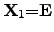and the thermodynamic driving forceis expressed as,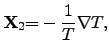(2.61)

where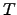has been identified as the absolute temperature by CARNOT2.20 [61,85], the corresponding equation system can be formulated with independent equations as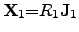(2.62)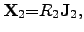(2.63)

where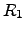and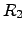are the electrical resistivity and the thermal heat resistance'', respectively. The heat resistance is also called thermal resistance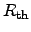in this thesis. The quantities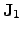and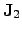are the electrical and the thermal current, respectively. The thermal current densityis also called heat flow density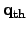. Several thermodynamic experiments over the last 150 years have shown that the electrical current is not independent of the temperature. Therefore, equations (2.62) and (2.63) are coupled. Introducing the standard notation, these equations can be adapted by cross coefficients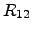and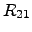and represent the ONSAGER relations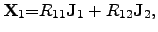(2.64)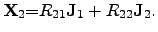(2.65)

For this equation system, THOMSON proposed the relation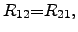(2.66)

which is also called reciprocity theorem'' of the ONSAGER relations. However, (2.66) implies that this relation follows from symmetric principles of thermodynamic theory. Hence, the reciprocity theorem neglects the loss during heat conduction and energy conversion and relation (2.66) assumes a balanced energy flow between the two subsystems. Thus, a steady stage is assumed with the request of (2.66) , where equilibrium conditions are applicable only within short range.

The principle of microscopic reversibility in (2.66) is less general than the second fundamental law of thermodynamics . For further investigated coupled systems, the currents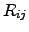may have different signs due to the different directions of the energy flows. Therefore, (2.66) is not sufficient enough to fulfill the second law of thermodynamics. Hence, the necessary condition for the equation system consisting of (2.64) and (2.65) to guarantee the second law with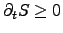yields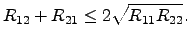(2.67)

This necessary condition has been originally proposed by BOLTZMANN in 1887 .

Writing the ONSAGER relations (2.64) and (2.65) as functions of driving forces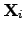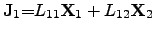(2.68)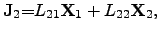(2.69)

where the necessary condition of type (2.67) remains valid accordingly for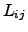as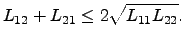(2.70)

To consider the ONSAGER relations in terms of energy, (2.68) and (2.69) can be multiplied by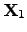and, respectively, leading to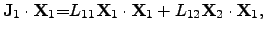(2.71)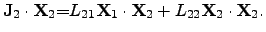(2.72)

These equations represent the products of the driving forcesand displacements of types of flow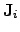. The result of (2.71) and (2.72) can be described as the dissipated energy per volume and per time and reads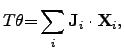(2.73)

where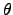is the entropy generation rate per unit volume and follows from the second law of thermodynamics (2.35)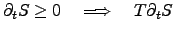(2.74)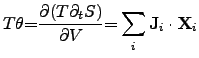(2.75)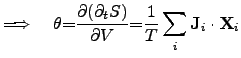(2.76)

where the entropy generation rate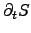can be determined by the sum of the power densities of all contributing subsystems. Hereby, the parts of the sums can be identified as the power densities of the participating systems which are determined by chemical reactions, the power loss due to heat transfer and JOULE's self-heating, and the power loss due to diffusion processes. The power density of chemical reactions can be expressed by scalar-valued quantities as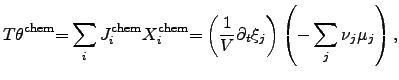(2.77)

where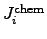is determined by the chemical reaction rate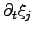per unit volume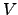. The chemical driving force is represented by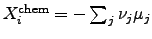, where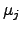denotes the chemical potential and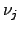the stoichiometric coefficient of the participating atom. The electrical power density can be identified by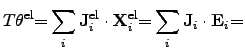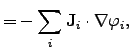(2.78)

where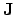and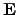are the electrical current density and the electrical field, respectively. The electric field can be expressed by the spatial gradient of an electrical potential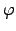. For electro-magnetical subsystems, the power density has to be appropriately adapted as discussed in Section 2.2.3. Another important contribution to the global entropy increase is the power loss due to thermal heat flow, which can be expressed in terms of (2.73) by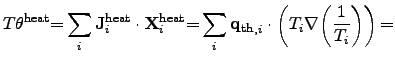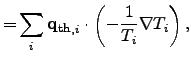(2.79)

whererepresents the local heat flux density. The second term in (2.79) depicts the thermal driving force according to FOURIER's empirical law. For diffusion processes, the power density can be identified as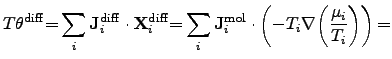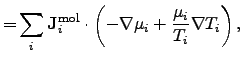(2.80)

where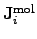is the mole number per unit area and time of the contributing species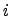. The driving force of diffusion processes is determined by the gradient of the chemical potential and by the gradient of the temperature.

Since the power density of diffusion processes has been determined for no external forces, an extension for the applied electrical field has to be made by introducing an additional term that depicts the force acting on charged particles inside the simulation domain. Hence, equation (2.80) has to be modified as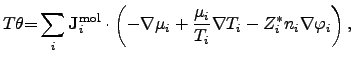(2.81)

where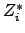is the effective valence charge of the species,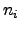is the species concentration per mole, and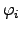is the corresponding electrical potential.

To conclude ONSAGER's thermodynamical treatment, the overall power density is thus given by the sum of the power densities of all contributing subsystems as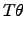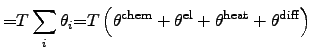(2.82)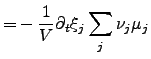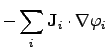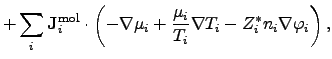(2.83)

where the thermodynamic power density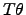is determined by the contributing chemical reactions, the electrical burden, heat flows, and molar diffusion processes.

Stefan Holzer 2007-11-19